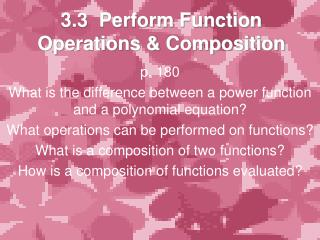DownloadDownload Presentation3.3 Perform Function Operations & Composition

# 3.3 Perform Function Operations & Composition

Télécharger la présentation## 3.3 Perform Function Operations & Composition

- - - - - - - - - - - - - - - - - - - - - - - - - - - E N D - - - - - - - - - - - - - - - - - - - - - - - - - - -
##### Presentation Transcript

1. 3.3 Perform Function Operations & Composition p. 180 What is the difference between a power function and a polynomial equation? What operations can be performed on functions? What is a composition of two functions? How is a composition of functions evaluated?

2. Operations on Functions: for any two functions f(x) & g(x) • Addition h(x) = f(x) + g(x) • Subtraction h(x) = f(x) – g(x) • Multiplication h(x) = f(x)*g(x) OR f(x)g(x) • Division h(x) = f(x)/g(x) OR f(x) ÷ g(x) • Composition h(x) = f(g(x)) OR g(f(x)) ** Domain – all real x-values that “make sense” (i.e. can’t have a zero in the denominator, can’t take the even nth root of a negative number, etc.)

3. Power Functions

4. Ex: Let f(x)=3x1/3 & g(x)=2x1/3. Find (a) the sum, (b) the difference, and (c) the domain for each. • 3x1/3 + 2x1/3 = 5x1/3 • 3x1/3 – 2x1/3 = x1/3 • Domain of (a) all real numbers Domain of (b) all real numbers

5. The functions fand geach have the same domain: all nonnegative real numbers. So, the domains of f + gand f – galso consist of all nonnegative real numbers. a. f(x) + g(x) f(x) – g(x) b. c. the domains of f + gand f – g Letf (x)= 4x1/2 andg(x)=–9x1/2. Find the following. SOLUTION f (x) + g(x) = [4 + (–9)]x1/2 = –5x1/2 SOLUTION = 4x1/2 – (–9x1/2) = [4 – (–9)]x1/2 = 13x1/2 f (x) – g(x)

6. Types Domains • All real numbers – if you can use positive numbers, negative numbers, or zero in the beginning functions and the result of combining functions. • All non-negative numbers -- if you can use positive numbers and zero in the beginning functions and the result of combining functions. • All positive numbers -- if you can use only positive numbers in the beginning function and the result of combining functions

7. Ex: Let f(x)=4x1/3 & g(x)=x1/2. Find (a) the product, (b) the quotient, and (c) the domain for each. • 4x1/3 * x1/2 = 4x1/3+1/2 = 4x5/6 = 4x1/3-1/2 = 4x-1/6 = (c) Domain of (a) all reals ≥ 0, because you can’t take the 6th root of a negative number (Non-neg #’s). Domain of (b) all reals > 0, because you can’t take the 6th root of a negative number and you can’t have a denominator of zero (Positive #’s).

8. The domain of f consists of all real numbers, and the domain of gconsists of all nonnegative real numbers. Because g(0) = 0, the domain of is restricted to all positive real numbers. f (x) f (x) 6x g(x) g(x) x3/4 = = 6x1/4 = 6x(1 – 3/4) Let f (x)= 6xand g(x) = x3/4. Find the following. SOLUTION SOLUTION the domain of

9. f (x) + g(x) f (x) – g(x) Try it… Let f (x) = –2x2/3 and g(x) = 7x2/3. Find the following. SOLUTION f (x) + g(x) = (–2 + 7)x2/3 = –2x2/3 + 7x2/3 = 5x2/3 SOLUTION f (x) – g(x) = [–2 + ( –7)]x2/3 = –2x2/3 – 7x2/3 = –9x2/3 The domains of f and g have the same domain: all non-negative real numbers. So , the domain of f + g and f – g also consist of all non-negative real numbers.

10. Composition of Functions

11. Ex: Let f(x)=2x-1 & g(x)=x2-1. Find (a) f(g(x)), (b) g(f(x)), (c) f(f(x)), and (d) the domain of each. (a) 2(x2-1)-1 = (c) 2(2x-1)-1 = 2(2-1x) = (b) (2x-1)2-1 = 22x-2-1 = (d)Domain of (a) all reals except x=±1. Domain of (b) all reals except x=0. Domain of (c) all reals except x=0, because 2x-1 can’t have x=0.

12. g(f(5)) ANSWER So, the value of g(f(5)) is 98. Let f(x) = 3x – 8 and g(x) = 2x2. Find the following. SOLUTION To evaluate g(f(5)), you first must find f(5). = 3(5) – 8 = 7 f(5) = 2(49) = 98. Theng( f(3)) = 2(7)2 = g(7)

13. f(g(5)) ANSWER So, the value of f( g(5)) is 142. Let f(x) = 3x – 8 and g(x) = 2x2. Find the following. SOLUTION To evaluate f(g(5)), you first must find g(5). g (5) = 2(5)2 =2(25) =50 Thenf( g(5)) = f(50) = 3(50) – 8 = 150 – 8 = 142.

14. Let f(x) = 2x–1 and g(x) = 2x + 7. Find f(g(x)),g(f(x)), and f(f(x)). Then state the domain of each composition. 2 2x + 7 = = = = f(2x+ 7) f(2x–1) f(2x–1) 4 = + 7 x SOLUTION f(g(x)) = 2(2x+ 7)–1 g(f(x)) = 2(2x–1) + 7 =4x–1 + 7 f(f(x)) = x = 2(2x–1)–1 The domain of f(g(x )) consists of all real numbers except x = –3.5. The domain of g(f(x)) consists of all real numbers except x = 0.

15. What is the difference between a power function and a polynomial equation? The power tells you what kind of equation—linear, quadratic, cubic… • What operations can be performed on functions? Add, subtract, multiply, divide. • What is a composition of two functions? A equation (function) is substituted in for the x in another equation (function). • How is a composition of functions evaluated? Write the outside function and substitute the other function for x.

16. Assignment Page 184, 3-27 every 3rd problem, 29-35 odd